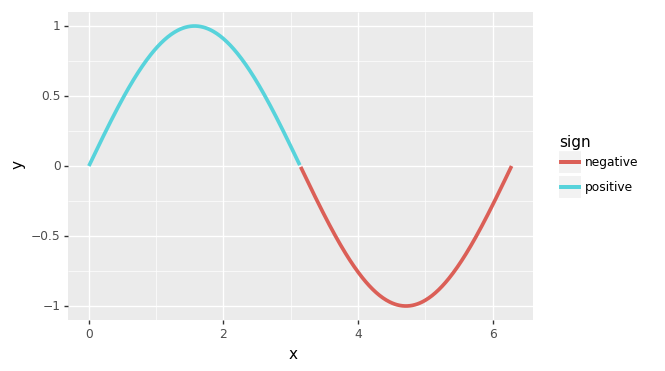# plydata¶

plydata is a library that provides a grammar for data manipulation. The grammar consists of verbs that can be applied to pandas dataframes or database tables. It is based on the R packages dplyr, tidyr and forcats. plydata uses the `>>` operator as a pipe symbol, alternatively there is the `ply(data, *verbs)` function that you can use instead of `>>`.

At present the only supported data store is the pandas dataframe. We expect to support sqlite and maybe postgresql and mysql.

## Example¶

```import numpy as np
import pandas as pd
from plydata import define, query, if_else, ply

# NOTE: query is the equivalent of dplyr's filter but with
#      slightly different python syntax  for the expressions

df = pd.DataFrame({
'x': [0, 1, 2, 3],
'y': ['zero', 'one', 'two', 'three']})

df >> define(z='x')
"""
x      y  z
0  0   zero  0
1  1    one  1
2  2    two  2
3  3  three  3
"""

df >> define(z=if_else('x > 1', 1, 0))
"""
x      y  z
0  0   zero  0
1  1    one  0
2  2    two  1
3  3  three  1
"""

# You can pass the dataframe as the # first argument
query(df, 'x > 1')  # same as `df >> query('x > 1')`
"""
x      y
2  2    two
3  3  three
"""

# You can use the ply function instead of the >> operator
ply(df,
define(z=if_else('x > 1', 1, 0)),
query('z == 1')
)
"""
x      y  z
2  2    two  1
3  3  three  1
"""
```

plydata piping works with plotnine.

```from plotnine import ggplot, aes, geom_line

df = pd.DataFrame({'x': np.linspace(0, 2*np.pi, 500)})
(df
>> define(y='np.sin(x)')
>> define(sign=if_else('y >= 0', '"positive"', '"negative"'))
>> (ggplot(aes('x', 'y'))
+ geom_line(aes(color='sign'), size=1.5))
)
```